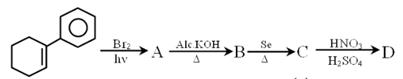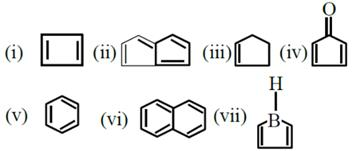Courses

# JEE Main Chemistry Mock - 8

## 25 Questions MCQ Test Mock Test Series for JEE Main & Advanced 2022 | JEE Main Chemistry Mock - 8

Description
This mock test of JEE Main Chemistry Mock - 8 for JEE helps you for every JEE entrance exam. This contains 25 Multiple Choice Questions for JEE JEE Main Chemistry Mock - 8 (mcq) to study with solutions a complete question bank. The solved questions answers in this JEE Main Chemistry Mock - 8 quiz give you a good mix of easy questions and tough questions. JEE students definitely take this JEE Main Chemistry Mock - 8 exercise for a better result in the exam. You can find other JEE Main Chemistry Mock - 8 extra questions, long questions & short questions for JEE on EduRev as well by searching above.
QUESTION: 1

Solution:
QUESTION: 2

Solution:
QUESTION: 3

### The pH of blood is around

Solution:
QUESTION: 4
The rate, at which a substance reacts at constant temperature, depends upon its
Solution:
QUESTION: 5
What will be the weight of deposited silver on passing 965 coulombs of electricity in solution of AgNO₃?
Solution:
QUESTION: 6
The indicator that is obtained by coupling the diazonium salt of sulphanilic acid with N,N-dimethylaniline is
Solution:
QUESTION: 7
For the reaction A→B, the rate law expression is rate = k[A]. Which of the following statements is incorrect?
Solution:
QUESTION: 8
Which of the following is an extensive property?
Solution:
QUESTION: 9
AgCl is soluble in NH₄OH solution. The solubility is due to formation of
Solution:
QUESTION: 10
The name of the element with atomic number 100 was adopted in honour of
Solution: Fermium (symbol Fm and atomic number 100) was named for the American physicist Enrico Fermi. It is a synthetic, radioactive, metallic transuranic element of the actinide series.
QUESTION: 11
Which one of the following is the strongest acid?
Solution:
QUESTION: 12
Corrosion can be prevented by
Solution:
QUESTION: 13
Which one of the following can be considered as weak electrolyte ?
Solution:
QUESTION: 14
A mixture of a weak acid (say acetic acid )and its salt with a strong base (say sodium acetate )is a buffer solution.Which other pair of substances from the following may have a similar property ?
Solution:
QUESTION: 15

Which of the following is not true in case of reaction with heated copper at 300oC?

Solution:
QUESTION: 16

Which of the following is a Lewis acid?

Solution:

"Lewis Acid is something that accepts a pair of electrons."

"A Lewis Base is something that donates a pair of electrons."

So Cl- would be a Lewis base.

NH3 is a Lewis Base.

BF3 is NOT a Lewis Base because it has an empty p orbital, this is a Lewis Acid.

C2H5OH is ethanol and that is a Lewis Base because the Oxygen has two lone pairs of electrons that it can donate.

QUESTION: 17
Orlon is a polymer of
Solution:
QUESTION: 18
Ethyl bromide treatements with alcoholic KOH gives
Solution:
QUESTION: 19

Which does not contain conjugate system?

Solution:

In dienes, when the double bonds connected to each other by a single bond, this arrangement is known as conjugated.

QUESTION: 20
Which one of the following solutions of sulphuric acid will exactly neutralise 25 ml of 0.2 M sodium hydroxide solution ?
Solution:
*Answer can only contain numeric values
QUESTION: 21

Sum of number of ions in aqueous solution of CrCl3.5NH3 and CrCl3 . 4NH3.

Solution:
*Answer can only contain numeric values
QUESTION: 22

Find the planner species out of the following species
SF2, SF4, SF6 , SO2, SO3

Solution:
*Answer can only contain numeric values
QUESTION: 23if ‘x’ is total number of benzene ring in compound(C) and ‘y’ is the total number of degree of unsaturation in compound (D) then the value of (x + y) is

Solution:
*Answer can only contain numeric values
QUESTION: 24

Identify total number of compound (s) which are unstable at room temperature ?Solution:
*Answer can only contain numeric values
QUESTION: 25

27 gm of Al react with excess of oxygen to give 4.95 gm of Al2O3, calculate % yield of reaction.

Solution:

Acidic oxide = CO2, SiO2
x = 2
Neutral oxide = CO
y = 1
2 + 1 = 3